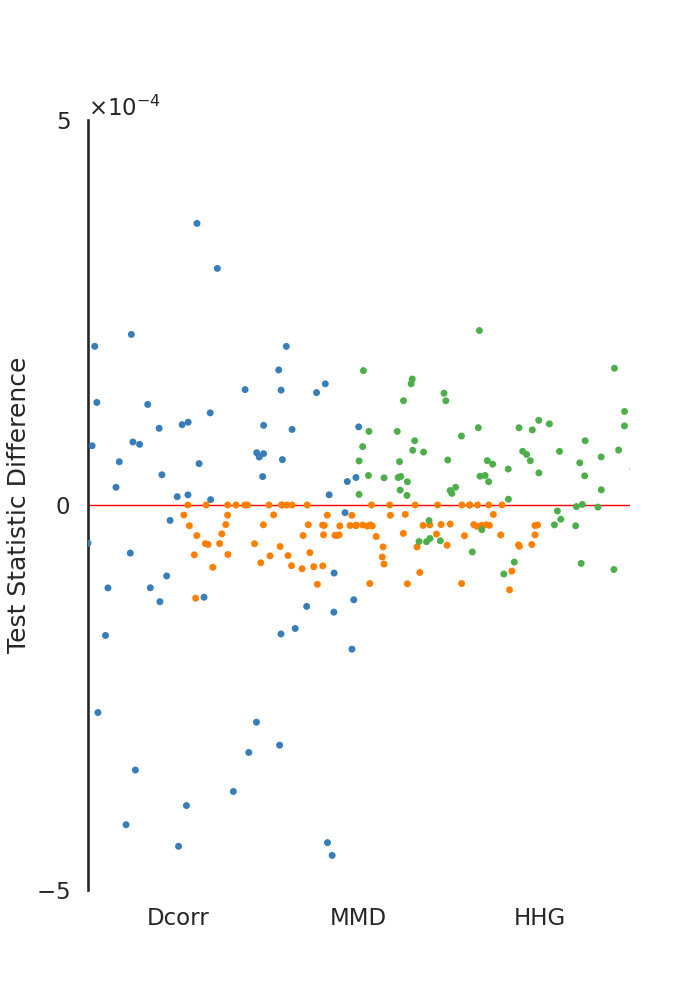# Comparisons of Test Statistics¶

There are few implementations in hyppo.independence the have implementations in R. Here, we compare the test statistics between the R-generated values and our package values. As you can see, there is a minimum difference between test statistics.

import os
import sys

import matplotlib.pyplot as plt
import matplotlib.ticker as mticker
import numpy as np
import pandas as pd
import seaborn as sns
from hyppo.independence import HHG, Dcorr
from hyppo.ksample import MMD
from hyppo.tools import rot_ksamp, spiral

sys.path.append(os.path.realpath(".."))

# make plots look pretty
sns.set(color_codes=True, style="white", context="talk", font_scale=1)
PALETTE = sns.color_palette("Set1")
sns.set_palette(PALETTE[1:])

# constants
N = 100  # number of replications to show
FONTSIZE = 20

# tests
TESTS = {
"Dcorr": Dcorr,
"MMD": MMD,
"HHG": HHG,
}

# generate the simulations, uncomment this code to regenerate
# for i in range(N):
#     x, y = spiral(1000, 1, noise=True)
#     sim = np.hstack([x, y])
#     np.savetxt("../benchmarks/same_stat/indep/{}.csv".format(i + 1), sim, delimiter=",")
#     sim1, sim2 = rot_ksamp("spiral", 200, 1, noise=True)
#     np.savetxt(
#         "../benchmarks/same_stat/ksample/sim1_{}.csv".format(i + 1), sim, delimiter=","
#     )
#     np.savetxt(
#         "../benchmarks/same_stat/ksample/sim2_{}.csv".format(i + 1), sim, delimiter=","
#     )

# compute test statistics, uncomment to recompute
# for key, test in TESTS.items():
#     stats = []
#     for i in range(N):
#         if key == "MMD":
#             sim1 = np.genfromtxt(
#                 "../benchmarks/same_stat/ksample/sim1_{}.csv".format(i + 1),
#                 delimiter=",",
#             )
#             sim2 = np.genfromtxt(
#                 "../benchmarks/same_stat/ksample/sim2_{}.csv".format(i + 1),
#                 delimiter=",",
#             )
#             stat = test(bias=True).statistic(sim1, sim2)
#         else:
#             sim = np.genfromtxt(
#                 "../benchmarks/same_stat/indep/{}.csv".format(i + 1), delimiter=","
#             )
#             x, y = np.hsplit(sim, 2)
#             stat = test().statistic(x, y)
#         stats.append(stat)
#     np.savetxt("../benchmarks/same_stat/{}.csv".format(key), stats, delimiter=",")

def plot_stats():
_ = plt.figure(figsize=(7, 10))
ax = plt.subplot(111)

test_names = list(TESTS.keys())
data = np.zeros((N, len(test_names)))
for i in range(len(test_names)):
if test_names[i] == "MMD":
hyppo_stat = np.genfromtxt("../benchmarks/same_stat/MMD.csv", delimiter=",")
r_stat = np.genfromtxt("../benchmarks/same_stat/RMMD.csv", delimiter=",")
else:
hyppo_stat = np.genfromtxt(
"../benchmarks/same_stat/{}.csv".format(test_names[i]), delimiter=","
)
r_stat = np.genfromtxt(
"../benchmarks/same_stat/R{}.csv".format(test_names[i]), delimiter=","
)
if (
test_names[i] == "HHG"
):  # Fix for large HHG stats so difference is comparable
hyppo_stat *= 1e-8
r_stat *= 1e-8
data[:, i] = np.abs(hyppo_stat) - np.abs(r_stat)

data = pd.DataFrame(data=data, columns=test_names)
sns.stripplot(
data=data,
edgecolor="gray",
size=5,
palette=["#377eb8", "#ff7f00", "#4daf4a"],
jitter=1,
)
ax.axhline(y=0, color="red", linewidth=1)

ax.spines["top"].set_visible(False)
ax.spines["right"].set_visible(False)
ax.spines["bottom"].set_visible(False)
ax.set_ylabel("Test Statistic Difference")
ax.set_ylim(-5e-4, 5e-4)
ax.set_yticks([-5e-4, 0, 5e-4])
f = mticker.ScalarFormatter(useOffset=False, useMathText=True)
f.set_powerlimits((-2, 2))
ax.yaxis.set_major_formatter(f)

# plot the statistic differences
plot_stats()Out:

/opt/buildhome/python3.7/lib/python3.7/site-packages/seaborn/cm.py:1582: UserWarning: Trying to register the cmap 'rocket' which already exists.
mpl_cm.register_cmap(_name, _cmap)
/opt/buildhome/python3.7/lib/python3.7/site-packages/seaborn/cm.py:1583: UserWarning: Trying to register the cmap 'rocket_r' which already exists.
mpl_cm.register_cmap(_name + "_r", _cmap_r)
/opt/buildhome/python3.7/lib/python3.7/site-packages/seaborn/cm.py:1582: UserWarning: Trying to register the cmap 'mako' which already exists.
mpl_cm.register_cmap(_name, _cmap)
/opt/buildhome/python3.7/lib/python3.7/site-packages/seaborn/cm.py:1583: UserWarning: Trying to register the cmap 'mako_r' which already exists.
mpl_cm.register_cmap(_name + "_r", _cmap_r)
/opt/buildhome/python3.7/lib/python3.7/site-packages/seaborn/cm.py:1582: UserWarning: Trying to register the cmap 'icefire' which already exists.
mpl_cm.register_cmap(_name, _cmap)
/opt/buildhome/python3.7/lib/python3.7/site-packages/seaborn/cm.py:1583: UserWarning: Trying to register the cmap 'icefire_r' which already exists.
mpl_cm.register_cmap(_name + "_r", _cmap_r)
/opt/buildhome/python3.7/lib/python3.7/site-packages/seaborn/cm.py:1582: UserWarning: Trying to register the cmap 'vlag' which already exists.
mpl_cm.register_cmap(_name, _cmap)
/opt/buildhome/python3.7/lib/python3.7/site-packages/seaborn/cm.py:1583: UserWarning: Trying to register the cmap 'vlag_r' which already exists.
mpl_cm.register_cmap(_name + "_r", _cmap_r)
/opt/buildhome/python3.7/lib/python3.7/site-packages/seaborn/cm.py:1582: UserWarning: Trying to register the cmap 'flare' which already exists.
mpl_cm.register_cmap(_name, _cmap)
/opt/buildhome/python3.7/lib/python3.7/site-packages/seaborn/cm.py:1583: UserWarning: Trying to register the cmap 'flare_r' which already exists.
mpl_cm.register_cmap(_name + "_r", _cmap_r)
/opt/buildhome/python3.7/lib/python3.7/site-packages/seaborn/cm.py:1582: UserWarning: Trying to register the cmap 'crest' which already exists.
mpl_cm.register_cmap(_name, _cmap)
/opt/buildhome/python3.7/lib/python3.7/site-packages/seaborn/cm.py:1583: UserWarning: Trying to register the cmap 'crest_r' which already exists.
mpl_cm.register_cmap(_name + "_r", _cmap_r)


The following shows the code that was used to compute the R test statistics. Certain lines were commented out depending on whether or not they were useful to calculate a given test statistic.

rm(list=ls())

require("energy")
require("kernlab")
require("HHG")
# change to your file path using setwd to same_stat/indep and same_stat/ksample
# filepath =

times = seq(1, 20, by=1)
statistics <- list()
for (t in times){
# df <- read.csv(paste(filepath, "/", t, ".csv", sep=""), sep=",")
df1 <- read.csv(paste(filepath, "/sim1_", t, ".csv", sep=""), sep=",")
df2 <- read.csv(paste(filepath, "/sim2_", t, ".csv", sep=""), sep=",")
# x <- df[, 1]
# y <- df[, 2]
x <- df1[,]
y <- df2[,]
# stat <- bcdcor(x, y)
# Dx = as.matrix(dist((x), diag = TRUE, upper = TRUE))
# Dy = as.matrix(dist((y), diag = TRUE, upper = TRUE))
# stat <- hhg.test(Dx, Dy, nr.perm=0)\$sum.chisq
stat <- kmmd(x, y, ntimes=0)@mmdstats
statistics <- c(statistics, list(stat))
}

df <- data.frame(matrix(unlist(statistics), nrow=length(statistics), byrow=T), stringsAsFactors=FALSE)
write.csv(df, row.names=FALSE)


Total running time of the script: ( 0 minutes 0.214 seconds)

Gallery generated by Sphinx-Gallery